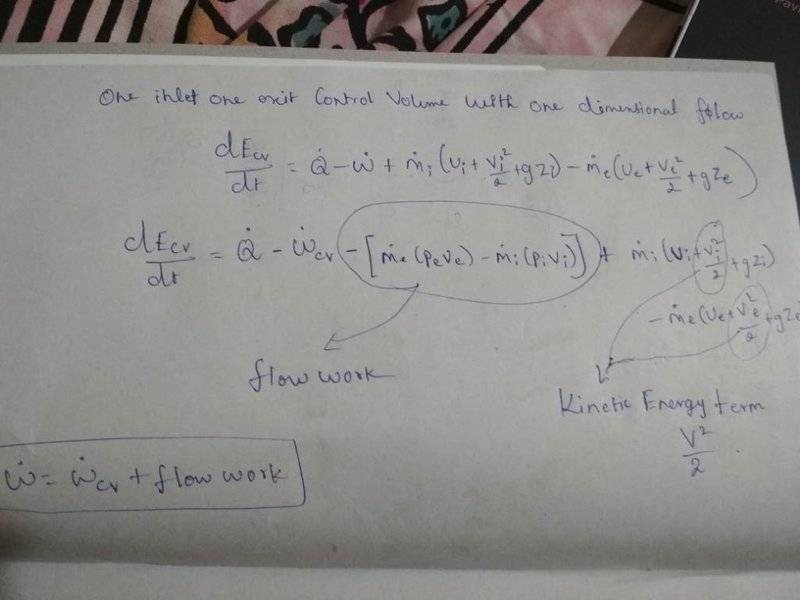# Are flow work and work done during isobaric expansion similar?

• Mohankpvk

#### Mohankpvk

In case of isobaric expansion, the system expands at constant pressure(the external pressure i.e. the resistance is constant.).In case of flow work, the fluid trying to exit the control volume, pushes against the pressure at the exit of the control volume or when entering the control volume, the surrounding pushes the fluid against the pressure at the inlet.Are these similar?

In case of isobaric expansion, the system expands at constant pressure(the external pressure i.e. the resistance is constant.).In case of flow work, the fluid trying to exit the control volume, pushes against the pressure at the exit of the control volume or when entering the control volume, the surrounding pushes the fluid against the pressure at the inlet.Are these similar?
I don't see the analogy, since, in the open system case, the inlet and outlet pressures are not necessarily the same.

•Mohankpvk
I don't see the analogy, since, in the open system case, the inlet and outlet pressures are not necessarily the same.
Ok.I just have another doubt. As per work energy principle, the energy spent in moving an object is stored as kinetic energy.Flow work is done to make the fluid move.In Bernouli's equation, why is it not included in kinetic energy (i.e) why kinetic energy and flow work are considered seperately?

Ok.I just have another doubt. As per work energy principle, the energy spent in moving an object is stored as kinetic energy.Flow work is done to make the fluid move.In Bernouli's equation, why is it not included in kinetic energy (i.e) why kinetic energy and flow work are considered seperately?
I don't understand what your asking. Can you write down an equation and identify the specific terms you are referring to?

I don't understand what your asking. Can you write down an equation and identify the specific terms you are referring to?
I took the equation in the photograph from "The fundamentals of thermodynamics" by Moran et. al. I have marked the flow work and the kinetic energy terms.My doubt is that if work done in moving the fluid will be stored as kinetic energy of the fluid then why K.E. and flow work are considered as two different terms?#### Attachments

The flow work represents the work required to push the fluid into and out of the control volume. This contributes to the changes in kinetic energy (as well as to other forms of energy included in the equation). Kinetic energy is not the only form of energy contained in the equation. There is also internal energy (of the fluid) and potential energy. In this open system form of the first law of thermodynamics, the next step is to lump the pv terms together with the internal energy entering and exiting to obtain the enthalpy of the flowing fluid entering and leaving.